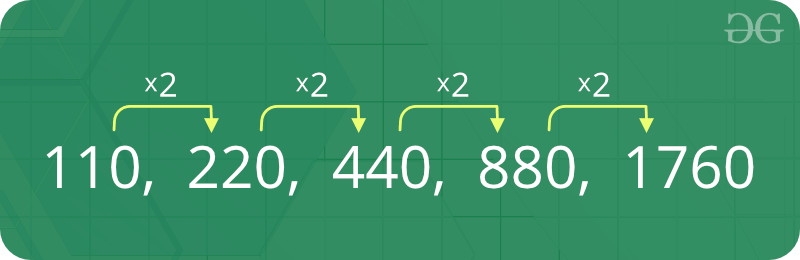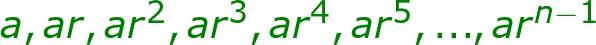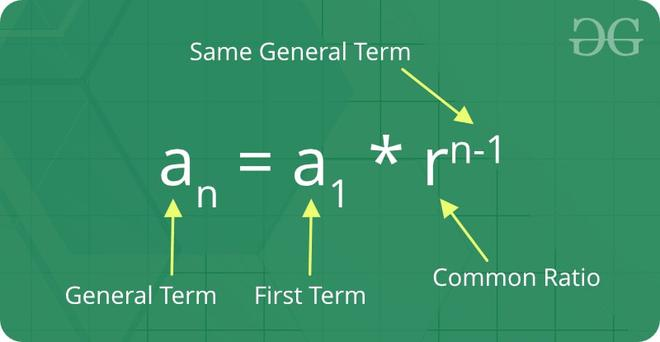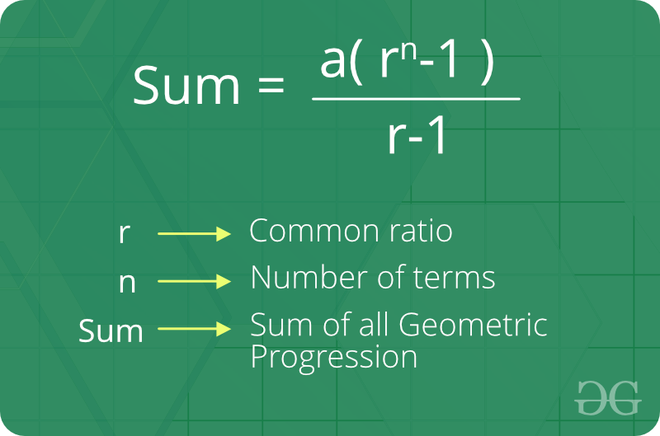# Geometric Progression

A sequence of numbers is called a Geometric progression if the ratio of any two consecutive terms is always the same.In simple terms, A geometric series is a list of numbers where each number, or term, is found by multiplying the previous term by a common ratio r. The general form of Geometric Progression is:GP-series

Where,
a = First term
r = common ratio
arn-1 = nth term

Example:

The sequence 2, 4, 8, 16 is a GP because ratio of any two consecutive terms in the series (common difference) is same (4 / 2 = 8 / 4 = 16 / 8 = 2).

### The geometric progression is of two types:

1. Finite geometric progression
2. Infinite geometric progression.

### 1. Finite geometric progression

In finite geometric progression contains a finite number of terms. The last term is always defined in this type of progression.

Example:

The sequence 1/2,1/4,1/8,1/16,…,1/32768 is a finite geometric series where the first term is 1/2 and the last term is 1/32768.

### 2. Infinite geometric progression

Infinite geometric progression contains an infinite number of terms.  The last term is not defined in this type of progression.

### Example:

Sequence 3, 9, 27, 81, … is an infinite series where the first term is 3 but the last term is not defined.

1. Initial term: In a geometric progression, the first number is called the initial term.
2. Common ratio: The ratio between a term in the sequence and the term before it is called the “common ratio.”
3. The behavior of a geometric sequence depends on the value of the common ratio. If the common ratio is:
• Positive, the terms will all be the same sign as the initial term.
• Negative, the terms will alternate between positive and negative.
• Greater than 1, there will be exponential growth towards positive or negative infinity (depending on the sign of the initial term).
• 1, the progression is a constant sequence.
• Between -1 and 1 but not zero, there will be exponential decay towards zero.
• -1, the progression is an alternating sequence.
• Less than -1, for the absolute values there is exponential growth towards (unsigned) infinity, due to the alternating sign.

### The formula for the nth term of a Geometric Progression:

If ‘a1′ is the first term and ‘r’ is the common ratio. Thus, the explicit formula for nth term of finite GP series:Nth term of a Geometric Progression

The formula for the sum of the nth term of Geometric Progression:Sum of the Nth term of Geometric Progression

How do we check whether a series is a Geometric progression or not?

The property of the GP series is that the ratio of the consecutive terms is same.

Approach:

1. First calculate the common ratio r by arr / arr
2. Iterate over an array and calculate the ratio of the consecutive terms.
3. Check if the calculated ratio is not equal to the common ratio r
• Return false
4. After traversal, if the calculated ratio is equal to the common ratio r every time
• Return true

Below is the implementation of the above approach:

## C++

 `// C++ program to check if a given array` `// can form geometric progression`   `#include `   `using` `namespace` `std;`   `bool` `is_geometric(``int` `arr[], ``int` `n)`   `{` `    ``if` `(n == 1)` `        ``return` `true``;`   `    ``// Calculate ratio` `    ``int` `ratio = arr / (arr);`   `    ``// Check the ratio of the remaining` `    ``for` `(``int` `i = 1; i < n; i++) {` `        ``if` `((arr[i] / (arr[i - 1])) != ratio) {` `            ``return` `false``;` `        ``}` `    ``}` `    ``return` `true``;` `}`   `// Driven Program` `int` `main()` `{` `    ``int` `arr[] = { 2, 6, 18, 54 };` `    ``int` `n = ``sizeof``(arr) / ``sizeof``(arr);`   `    ``(is_geometric(arr, n)) ? (cout << ``"True"` `<< endl)` `                           ``: (cout << ``"False"` `<< endl);`   `    ``return` `0;` `}`

## Java

 `// Java program to check if a given array` `// can form geometric progression` `import` `java.util.Arrays;`   `class` `GFG {`   `    ``// function to check series is` `    ``// geometric progression or not` `    ``static` `boolean` `is_geometric(``int` `arr[], ``int` `n)` `    ``{` `        ``if` `(n == ``1``)` `            ``return` `true``;`   `        ``// Calculate ratio` `        ``int` `ratio = arr[``1``] / (arr[``0``]);`   `        ``// Check the ratio of the remaining` `        ``for` `(``int` `i = ``1``; i < n; i++) {` `            ``if` `((arr[i] / (arr[i - ``1``])) != ratio) {` `                ``return` `false``;` `            ``}` `        ``}` `        ``return` `true``;` `    ``}`   `    ``// driver code` `    ``public` `static` `void` `main(String[] args)` `    ``{` `        ``int` `arr[] = { ``2``, ``6``, ``18``, ``54` `};` `        ``int` `n = arr.length;`   `        ``if` `(is_geometric(arr, n))` `            ``System.out.println(``"True"``);` `        ``else` `            ``System.out.println(``"False"``);` `    ``}` `}`

## Python3

 `def` `is_geometric(li):` `    ``if` `len``(li) <``=` `1``:` `        ``return` `True` `        `  `    ``# Calculate ratio` `    ``ratio ``=` `li[``1``]``/``float``(li[``0``])` `    `  `    ``# Check the ratio of the remaining` `    ``for` `i ``in` `range``(``1``, ``len``(li)):` `        ``if` `li[i]``/``float``(li[i``-``1``]) !``=` `ratio: ` `            ``return` `False` `    ``return` `True`   `print``(is_geometric([``2``, ``6``, ``18``, ``54``]))`

## C#

 `// C# program to check if a given array` `// can form geometric progression` `using` `System;`   `class` `Geeks {`   `    ``static` `bool` `is_geometric(``int``[] arr, ``int` `n)` `    ``{` `        ``if` `(n == 1)` `            ``return` `true``;`   `        ``// Calculate ratio` `        ``int` `ratio = arr / (arr);`   `        ``// Check the ratio of the remaining` `        ``for` `(``int` `i = 1; i < n; i++) {` `            ``if` `((arr[i] / (arr[i - 1])) != ratio) {` `                ``return` `false``;` `            ``}` `        ``}` `        ``return` `true``;` `    ``}`   `    ``// Driven Program` `    ``public` `static` `void` `Main(String[] args)` `    ``{` `        ``int``[] arr = ``new` `int``[] { 2, 6, 18, 54 };` `        ``int` `n = arr.Length;`   `        ``if` `(is_geometric(arr, n))` `            ``Console.WriteLine(``"True"``);` `        ``else` `            ``Console.WriteLine(``"False"``);` `    ``}` `}`

## PHP

 ``

## Javascript

 ``

Output

`True`

Time Complexity: O(n), Where n is the length of the given array.

Auxiliary Space: O(1)

Basic Program related to Geometric Progression

More problems related to Geometric Progression

Recent Articles on Geometric Progression!

Feeling lost in the world of random DSA topics, wasting time without progress? It's time for a change! Join our DSA course, where we'll guide you on an exciting journey to master DSA efficiently and on schedule.
Ready to dive in? Explore our Free Demo Content and join our DSA course, trusted by over 100,000 geeks!